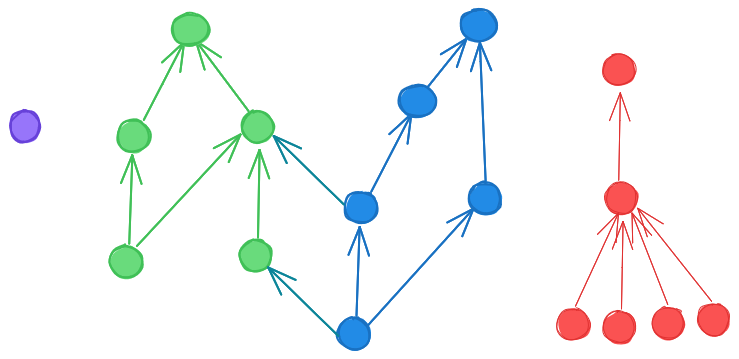# People’s choices determine a partial ordering over people’s desirability

Consider the following relationship:

$$\delta_{i}(a, b) = \begin{cases} -1 \text{ if person i has chosen person b over person a} \\ 0 \text{ if person i has not made a choice between person a and person b} \\ 1 \text{ if person i has chosen person a over person b} \end{cases}$$ $$a \le b \text{ iff } \sum_{i} \delta_{i}(a, b) < 0$$

### Is this a partial ordering?

For a relationship to be a partial ordering, it has to be the case that:

$$a \le a \text{ (Reflexivity)}$$ $$(a \le b) \land (b \le a) \implies a = b \text{ (Antisymmetry)}$$ $$(a \le b) \land (b \le c) \implies a \le c \text{ (Transitivity)}$$

Antisymmetry

We do get antisymmetry, since it can’t be the case that both of these are true at the same time:

$$\sum_{i=0}^n \delta_i(a, b) < 0$$ $$\sum_{i=0}^n \delta_i(b, a) = - \sum_{i=0}^n \delta_i(a, b) < 0$$

Reflexivity

We can also get reflexivity, by dictating that $$a \le a$$.

Transitivity

We don’t get transitivity for free, because you could have a rock-papers-scissors situation. For example, consider a situation where you have a, an attractive celebrity, b, the less attractive but still pretty cool friend of that celebrity, and c, the husband of a’s childhood sweetheart. Then:

• $$b \le a$$, because of the people who choose between a and b, they mostly choose a
• $$c \le b$$, because of the people who choose between b and c, they mostly choose b
• $$a \le c$$, because the only person who chooses between a and c is c’s wife, who chooses c

And if fact you do see this kind of thing in some animals.

With regard to their throat color, the males of the common side-blotched lizard can also be distinguished into three morphotypes which compete against each other for reproduction. Males with an orange-colored throat aggressively claim a large territory for themselves to build a harem. Meanwhile, the males with a blue-colored throat are less dominant, but guard their mating partner from other males. Finally, males with a yellow-colored throat mimic the female phenotype and rely on a sneaking strategy. From that polymorphism, a rock-paper-scissor like game emerges: the dominant orange-colored males take females from the blue-colored males by force, the blue-colored males are able to protect their females from the yellow-colored males, which in turn are able to sneak into the harem of orange-colored males. –Wikipedia, Alternative mating strategy: Common side-blotched lizard

Even worse, you might still have a situation where

• 70% of people are indifferent between a and b, and 30% prefer a
• 70% of people are indifferent between b and c, and 30% prefer b
• 70% of people are indifferent between c and a, and 30% prefer c

### Attaining transitivity

However, we might have the intuition that these types of loops are rare. And so we can define a second relationship:

$$\begin{eqnarray} a \le_2 b \text{ iff } \nonumber \\ &\text{ there exists some chain such that } a \le x_1 \le ... \le x_n \le b \text{, possibly just } a \le b \tag{1} \\ &\text{ AND there is no chain such that } b \le x_1 \le ... \le x_n \le a \tag{2} \\ &\text{ AND there is no chain such that } c \le y_1 \le ... \le y_l \le a \le x_1 \le ... \le x_m \le b \le z_1 \le ... \le z_n \le c \tag{3}\\ \end{eqnarray}$$

So basically, we synthetically force transitivity, but we remove the places where there are loops, to preserve antisymmetry.

Now, if $$a \le_2 b$$ and $$b \le_2 c$$, then $$a \le_2 c$$, because we can concatenate the chains from (1), and staring at the other two properties should also convince the reader that they are fulfilled.

### How does this look?

So now we have a partial ordering. There is no absolute highest or lowest point, but there is some notion of higher and lower:Blue and green might be two partially overlapping social groups, red a separate clique, and purple someone who married their childhood sweetheart.